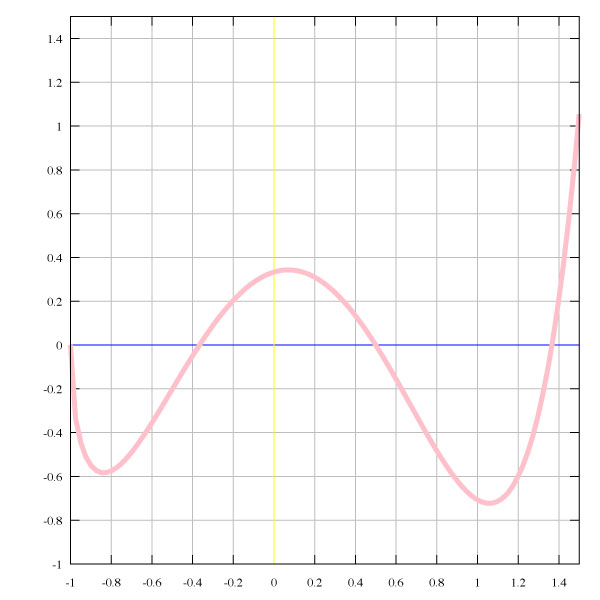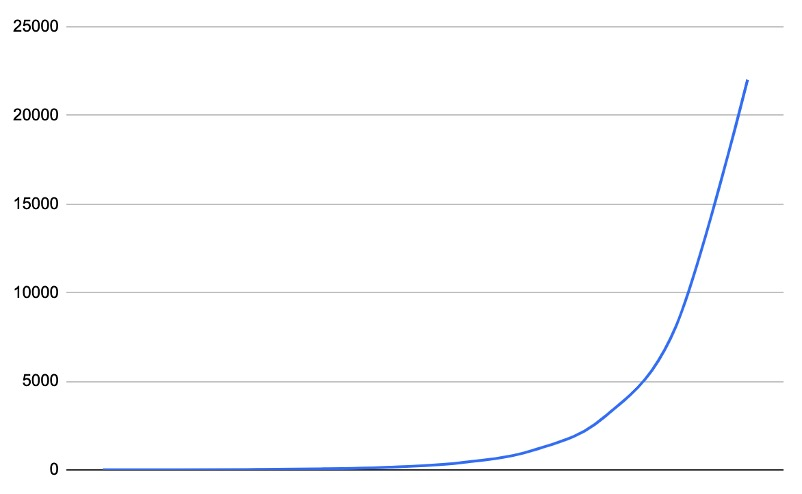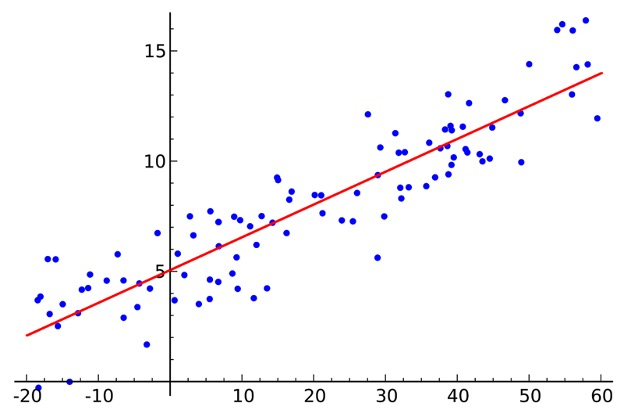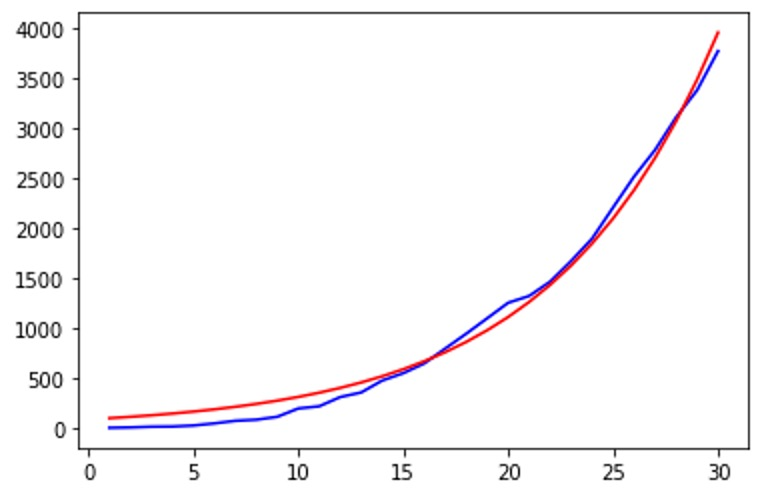Closescroll

# Approximation data by exponential function on Python

In today's world, the importance of conducting data science research is gaining momentum every day. This applies to so many aspects of the life of an individual, and of society as a whole. Accurate modeling of social, economic, and natural processes is vital.

One of the important processes in data analysis is the approximation process. If you correctly approximate the available data, then it becomes possible to estimate and predict future values. Thus, a weather forecast, a preliminary estimate of oil prices, economic development, social processes in society, and so on can be made. Most processes in nature are described by exponential functions. An exponential in Python is easily calculated by standard function from its mathematical library. Let's consider what exactly is a function and its approximation.

## What is a function?

The function (relation, operator, transformation) in mathematics determines the correspondence between the elements of two sets, established by such a rule that each element of the first set corresponds to one and only one element of the second set.

The mathematical concept of a function expresses an intuitive idea of how one value completely determines the value of another value.

Often, the term “function” refers to a numerical function, that is, a function that puts one number in correspondence with another.

More strictly, the function f maps the set X to the set Y. The function is also denoted by y = f (x).

In mathematics and data science, this is one of the fundamental concepts for computing and data analysis. The function can be represented in graphical form; for instance, in two dimensions.Image source

## An exponential function and why it is important in data science?

As stated earlier, a lot of processes can be described using an exponential function. The function y = Exp(x) is an exponential function with the base e = 2.718281828, i.e. Euler number. Exponential growth is an increase in value where the growth rate is proportional to the value of the quantity itself. Please take a look at the following table and graph to clearly understand the nature of exponential growth.

The Python exponential function is available in the math library and can be called as follows:

``````import math

math.exp(x)``````

xy = exp(x)
12.718281828
27.389056099
320.08553692
454.59815003
5148.4131591
6403.4287935
71096.633158
82980.957987
98103.083928
1022026.46579Please remember that the exponential function grows very fast with small increments of argument x and potentially can generate overflow. For instance when x=100, i.e. exp(100) will give us a very large number 2.6881171e+43.

## How to approximate a set of data by the exponential function

Approximation (lat. proxima - closest) is a scientific method consisting of replacing some objects with others, in a sense, close to the original, but simpler.

Approximation allows one to study the numerical characteristics and qualitative properties of an object, reducing the problem to the study of simpler or more convenient objects (for example, those whose characteristics are easily calculated or whose properties are already known).

You can approximate the input values using the approximation functions. The most commonly used approximation is linear, polynomial, and exponential.

## Non-linear least-squares problem

The least-squares method is the method of finding the optimal linear regression parameters, such that the sum of the squared errors (regression residuals) is minimal. The method consists of minimizing the Euclidean distance between two vectors, i.e. the vector of the restored values of the dependent variable and the vector of the actual values of the dependent variable.

This method very often is used for optimization and regression, as well as Python library scipy in method scipy.optimize.curve_fit () effectively implemented this algorithm. If we apply an exponential function and a data set x and y to the input of this method, then we can find the right exponent for approximation.Image source

## Python code for approximation example

Let's solve the problem of approximating a data set using an exponent. Of course, it is necessary to note that not all data can be approximated using an exponent, but in many cases when the law of change or function is exponential, this is quite possible.

For example, take data that describes the exponential increase in the spread of the virus. This data can be approximated fairly accurately by an exponential function, at least in pieces along the X-axis.

To do this, we will use the standard set from Python, the numpy library, the mathematical method from the sсipy library, and the matplotlib charting library.

The Python exp function from the numpy package helpssolve this task. The Numpy exponential function can be called the same way as in the math library, but it takes an array for input:

``````import numpy as np
in = [1, 2, 3, 4, 5]
out = np.exp(in)``````

To find the parameters of an exponential function of the form y = a * exp (b * x), we use the optimization method. To do this, the scipy.optimize.curve_fit () the function is suitable for us. This method uses a non-linear least squares algorithm to match the function that we specify at the input.

Exponential approximation is very popular in different areas of engineering, numerical methods, statistical applications, machine learning, and more. It allows you to make differentiation and integration in a very easy way.

This is one of the optimization methods, more details can be found here. If we find such a and b with which we can very similarly describe the law of the relationship x, y in the data, then we get the opportunity to build a function for other new values of the argument. This allows you to, predict the growth of the function for the following values along the X-axis, for example.

See the scipy.optimize.curve_fit () function manual for more details here

Now let's look at a small piece of Python code that:

1. Specifies input values for x, y
2. Using curve_fit(), calculate the value of a, b in an exponential function
3. An exponent function is defined as a lambda function lambda x1, a, b: a * numpy.exp (b * x1)
4. Then draw graphs of original data (blue), approximated data (red).
``````import numpy
from scipy.optimize import curve_fit
import matplotlib.pyplot as plt

x = numpy.arange(1, 31, 1)
y = numpy.array([3,7,14,16,26,47,73,84,113,196,218,310,356,475,548,645,794,
942,1096,1251,1319,1462,1668,1892,2203,2511,2777,3102,3372,3764])

[a, b], res1 = curve_fit(lambda x1,a,b: a*numpy.exp(b*x1),  x,  y)

y1 = a * numpy.exp(b * x)

plt.plot(x, y, 'b')
plt.plot(x, y1, 'r')
plt.show()``````This graph shows that the red curve (approximated data using the exponent) and the blue curve (real data) accurately describe the nature of the data change.

It is worth noting that you can get a sufficiently large value of the approximation error if your input data character obeys some other dependence that is different from the exponential one. In this case, the graph is divided into separate sections and you can try to approximate each section with its exponent. Or select another approximation function, for example, a polynomial.

## Conclusion

Concluding this article about data approximation using an exponential function, let’s note that now there are very good and effective tools for solving such an important problem. Using Python language and libraries like numpy and scipy, you can simply work wonders in data science, as shown in this task. The potential of approximation using an exponential function in the first approximation makes it possible to make predictions for a certain type of task in the economy, natural phenomena and in the social sphere. We clearly explained how to calculate the exponential function in Python and described methods of its approximation.

Our data science specialists are very well-trained in solving non-standard problems. Svitla Systems works with complex projects and has vast experience. We know how to satisfy customer requests, coordinate project requirements in agile mode, and maintain efficient communication.

by Svitla Team
June 03, 2020